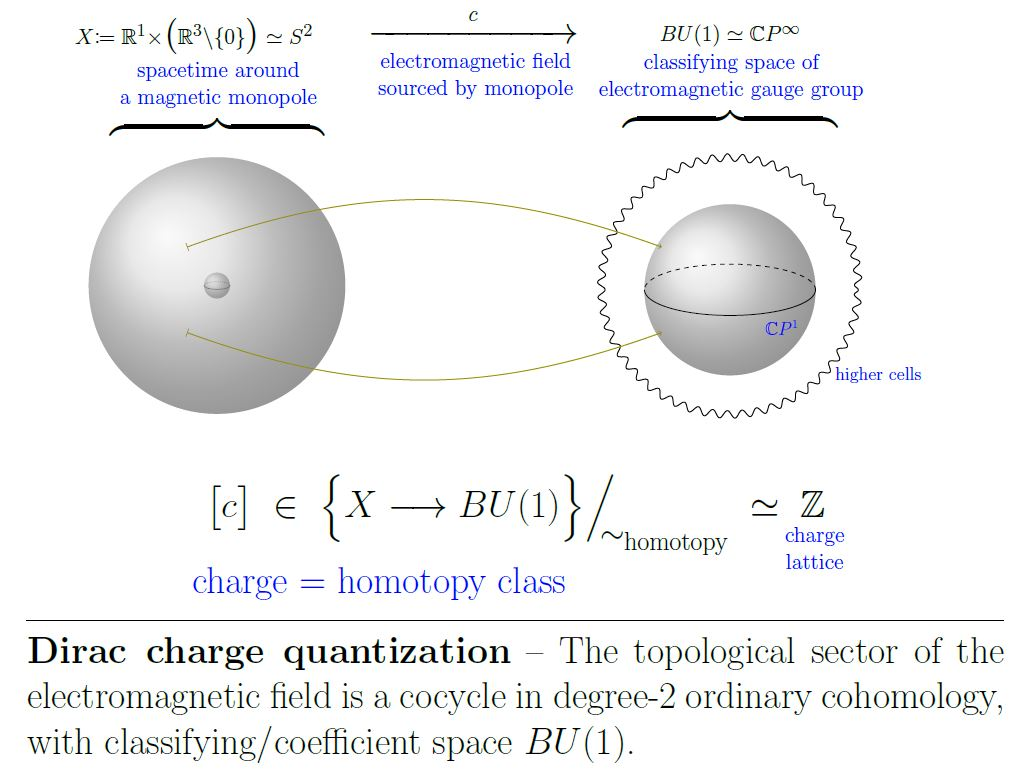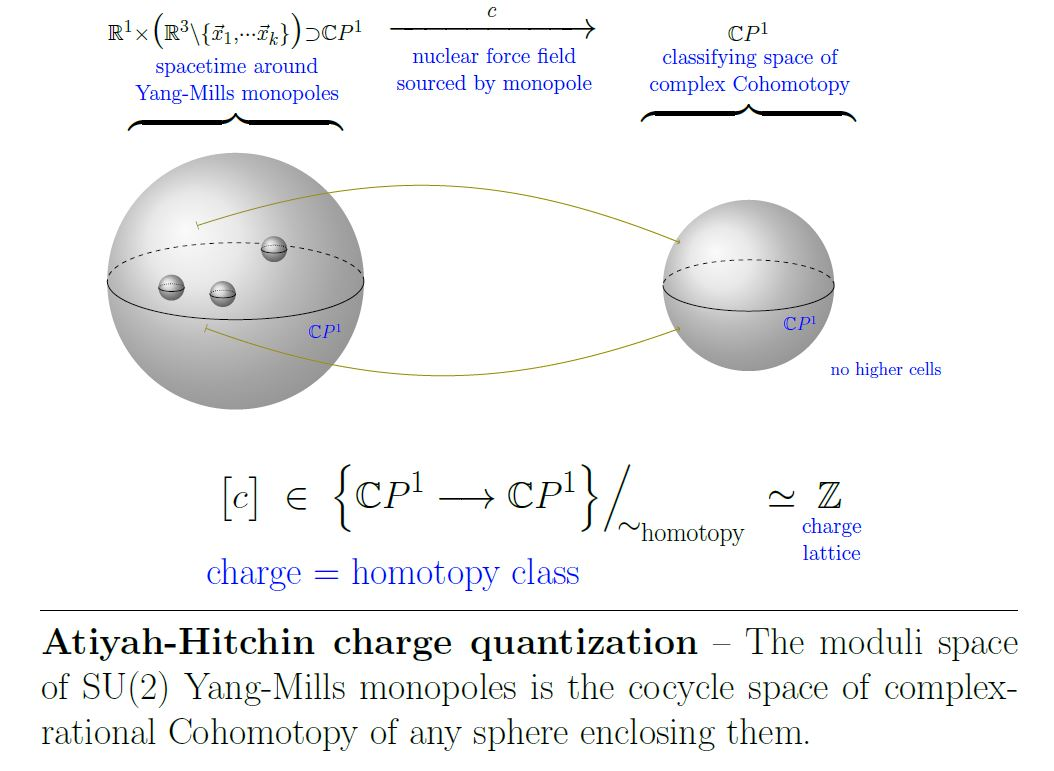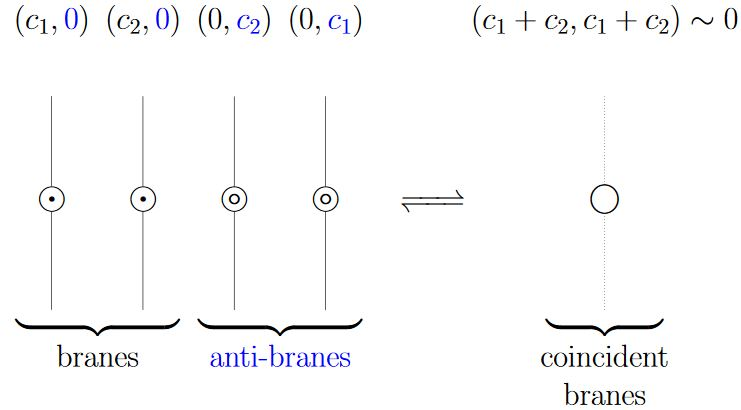# nLab Dirac charge quantization

Contents

## Surveys, textbooks and lecture notes

#### $\infty$-Chern-Weil theory

∞-Chern-Weil theory

∞-Chern-Simons theory

∞-Wess-Zumino-Witten theory

## Theorems

#### Differential cohomology

differential cohomology

# Contents

## Idea

### For monopole charges in electromagnetism

If the field of electromagnetism serves as a background gauge field for electrically charged quantum particles it is subject to a quantization condition: Outside the locus of any magnetic charge – for instance a magnetic monopole topological defect – the electromagnetic field must be a connection on a principal U(1) bundle whose first Chern class is the discrete measure for the units of magnetic charge. Equivalently this means that the electromagnetic field is a cocycle in ordinary differential cohomology of degree 2.

In the underlying topological sector (“monopole”/“instantons”-sector) this is integral cohomology in degree-2, whose classifying space is equivalently the infinite complex projective space $B U(1) \simeq \mathbb{C}P^\infty$:

$\,$This goes back to an insight due to Dirac 31. See Heras 18 for traditional elementary review, but see Alvarez 85, Frankel and Mangiarotti-Sardanashvily 00 for exposition of the modern picture in terms of fiber bundles in physics. See Freed 00, Section 2 for review in terms of differential cohomology with outlook to generalization to higher gauge fields in string theory (more on which in the references below).

A closely related phenomenon is the magnetic flux quantization in type II superconductors, see there.

On the locus of the magnetic charge itself the situation is more complex. There the magnetic current is given by a cocycle in ordinary differential cohomology of degree 3 (with compact support) and now the electromagnetic field is a connection on a twisted bundle (Freed 00, Section 2).

### For monopole charges in non-abelian Yang-Mills theory

A similar charge quantization condition govers monopoles in SU(2)-Yang-Mills theory, see at moduli space of monopoles. Here the Atiyah-Hitchin charge quantization (Atiyah-Hitchin 88, Theorem 2.10) says that the moduli space of monopoles is the complex-rational 2-Cohomotopy of an asymptotic 2-sphere enclosing the monopoles:

$\,$## Reference

See also the references at fiber bundles in physics.

### For the electromagnetic field

The original argument for charge quantization of the electromagnetic field is due to

Review:

See also

### For the weak nuclear force field

Discussion of the moduli space of monopoles for SU(2)-Yang-Mills theory (weak nuclear force):

### D-brane charge quantization in topological K-theory

On the conjectural D-brane charge quantization in topological K-theory:

#### Origin and basics

The idea that D-branes have Dirac charge quantization in topological K-theory originates with:

Here:From Sch 18

Expression of these D-brane K-theory classes via the Atiyah-Hirzebruch spectral sequence:

Specifically for D-branes in WZW models see

• Peter Bouwknegt, A note on equality of algebraic and geometric D-brane charges in WZW models (pdf)

Discussion of D-brane matrix models taking these K-theoretic effects into account (K-matrix model) is in

• T. Asakawa, S. Sugimoto, S. Terashima, D-branes, Matrix Theory and K-homology, JHEP 0203 (2002) 034 (arXiv:hep-th/0108085)

### Twisted, equivariant and differential refinement

Discussion of charge quantization in twisted K-theory for the case of non-vanishing B-field:

An elaborate proposal for the correct flavour of equivariant KR-theory needed for orientifolds is sketched in:

Discussion of full-blown twisted differential K-theory and its relation to D-brane charge in type II string theory

Discussion of full-blown twisted differential orthogonal K-theory and its relation to D-brane charge in type I string theory (on orientifolds):

#### Reviews

See also for instance

Discussion of D-branes in KK-theory is reviewed in

based on

In particular (BMRS2) discusses the definition and construction of D-brane charge as a generalized index in KK-theory. The discussion there focuses on the untwisted case. Comments on the generalization of this to topologicall non-trivial B-field and hence twisted K-theory is in

#### Conceptual problems

But there remain conceptual issues with the proposal that D-brane charge is in K-theory, as highlighted in

In particular, actual checks of the proposal that D-brane charge is given by K-theory, via concrete computation in boundary conformal field theory, have revealed some subtleties:

• Stefan Fredenhagen, Thomas Quella, Generalised permutation branes, JHEP 0511:004, 2005 (arXiv:hep-th/0509153)

It might surprise that despite all the progress that has been made in understanding branes on group manifolds, there are usually not enough D-branes known to explain the whole charge group predicted by (twisted) K-theory.

The closest available towards an actual check of the argument for K-theory via open superstring tachyon condensation (Witten 98, Section 3) seems to be

• Theodore Erler, Analytic Solution for Tachyon Condensation in Berkovits’ Open Superstring Field Theory, JHEP 1311 (2013) 007 (arXiv:1308.4400)

which, however, concludes (on p. 32) with:

It would also be interesting to see if these developments can shed light on the long-speculated relation between string field theory and the K-theoretic description of D-brane charge $[$75, 76, 77$]$. We leave these questions for future work.

See also

which still lists (on p. 112) among open problems of string field theory:

“Are there topological invariants of the open string star algebra representing D-brane charges?”

#### For orbifolds in equivariant K-theory

The proposal that D-brane charge on orbifolds is measured in equivariant K-theory (orbifold K-theory) goes back to

It was pointed out that only a subgroup of equivariant K-theory can be physically relevant in

Further discussion of equivariant K-theory for D-branes on orbifolds includes the following:

Discussion of real K-theory for D-branes on orientifolds includes the following:

The original observation that D-brane charge for orientifolds should be in KR-theory is due to

and was then re-amplified in

With further developments in

Discussion of orbi-orienti-folds using equivariant KO-theory is in

Discussion of the alleged K-theory classification of D-brane charge in relation to the M-theory C-field is in

See also

More complete discussion of double dimensional reduction of the supergravity C-field in 11d to the expected B-field and RR-field flux forms in 10d:

### For the C-field in M-theory

Discussion of shifted C-field flux quantization of the C-field in D=11 supergravity/M-theory:

Discussion in twisted Cohomotopy (“Hypothesis H”):

and in equivariant Cohomotopy:

Last revised on March 31, 2021 at 07:43:46. See the history of this page for a list of all contributions to it.